Documentation

### This is machine translation

Mouseover text to see original. Click the button below to return to the English version of the page.

## Train Reinforcement Learning Agent in Basic Grid World

This example shows how to solve a grid world environment using reinforcement learning by training Q-learning and SARSA agents. For more information on these agents, see Q-Learning Agents and SARSA Agents, respectively.

This grid world environment has the following configuration and rules:

1. A 5-by-5 grid world bounded by borders, with 4 possible actions (North=1, South=2, East=3, West=4).

2. The agent begins from cell [2,1] (second row, first column).

3. The agent receives reward +10 if it reaches the terminal state at cell [5,5] (blue).

4. The environment contains a special jump from cell [2,4] to cell [4,4] with +5 reward.

5. The agent is blocked by obstacles (black cells).

6. All other actions result in -1 reward.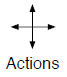### Create Grid World Environment

Create the basic grid world environment.

`env = rlPredefinedEnv("BasicGridWorld");`

To specify the initial state of the agent is always [2,1], specify a reset function that returns the initial agent state. This function is called at the start of each training episode and simulation. The states are numbered starting at position [1,1] and counting down the column. Therefore, create an anonymous function handle that sets the initial state to `2`.

`env.ResetFcn = @() 2;`

Fix the random generator seed for reproducibility.

`rng(0)`

### Create Q-Learning Agent

To create a Q-learning agent, first create a Q table using the observation and action specifications from the grid world environment. Set the learn rate of the representation to 1.

```qTable = rlTable(getObservationInfo(env),getActionInfo(env)); tableRep = rlRepresentation(qTable); tableRep.Options.LearnRate = 1;```

Next, create a Q-learning agent using this table representation, configuring the epsilon-greedy exploration. For more information on creating Q-learning agents, see `rlQAgent` and `rlQAgentOptions`.

```agentOpts = rlQAgentOptions; agentOpts.EpsilonGreedyExploration.Epsilon = .04; qAgent = rlQAgent(tableRep,agentOpts);```

### Train Q-Learning Agent

To train the agent, first specify the training options. For this example, use the following options:

• Train for at most 200 episodes, with each episode lasting at most 50 time steps.

• Stop training when the agent receives an average cumulative reward greater than 10 over 30 consecutive episodes.

For more information, see `rlTrainingOptions`.

```trainOpts = rlTrainingOptions; trainOpts.MaxStepsPerEpisode = 50; trainOpts.MaxEpisodes= 200; trainOpts.StopTrainingCriteria = "AverageReward"; trainOpts.StopTrainingValue = 11; trainOpts.ScoreAveragingWindowLength = 30;```

Train the Q-Learning agent using the `train` function. This may take several minutes to complete. To save time while running this example, load a pretrained agent by setting `doTraining` to `false`. To train the agent yourself, set `doTraining` to `true`.

```doTraining = false; if doTraining % Train the agent. trainingStats = train(qAgent,env,trainOpts); else % Load pretrained agent for the example. load('basicGWQAgent.mat','qAgent') end```

The Episode Manager window opens and displays the training progress.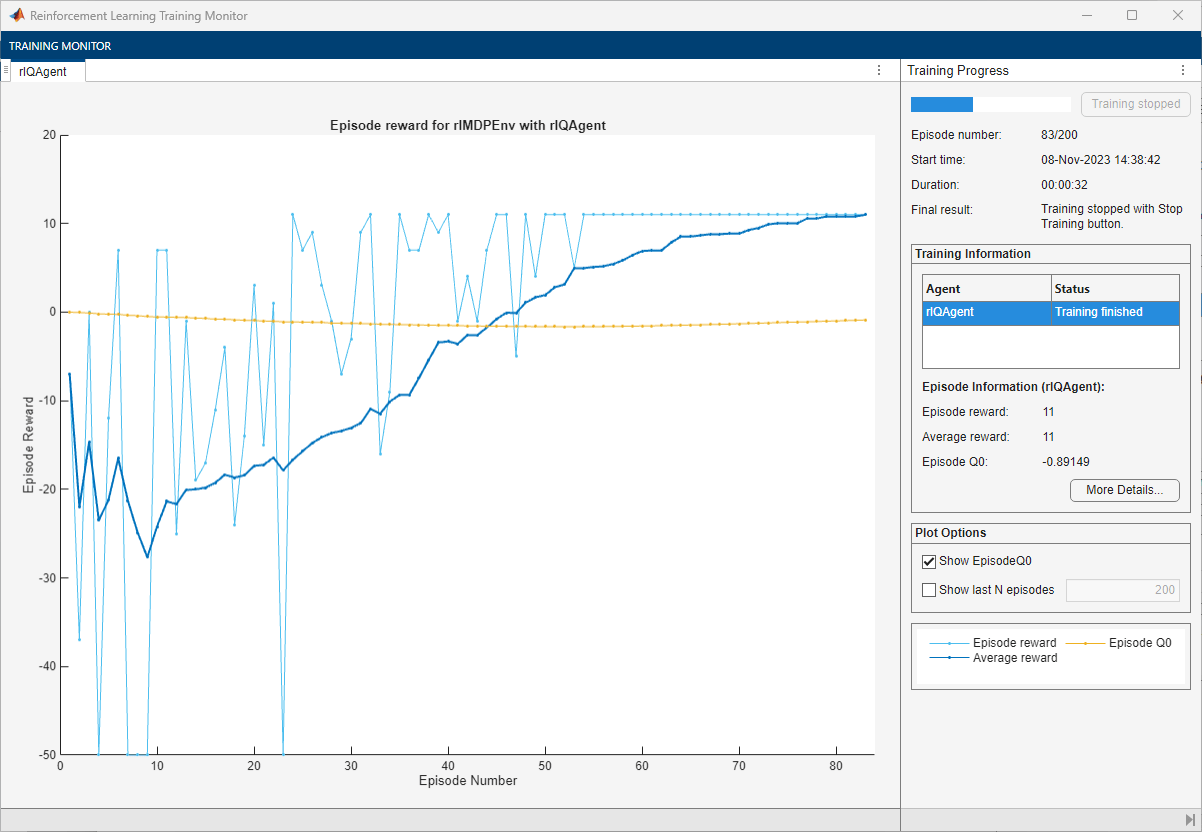### Validate Q-Learning Results

To validate the training results, simulate the agent in the training environment.

Before running the simulation, visualize the environment and configure the visualization to maintain a trace of the agent states.

```plot(env) env.Model.Viewer.ShowTrace = true; env.Model.Viewer.clearTrace;```

Simulate the agent in the environment using the `sim` function.

`sim(qAgent,env)`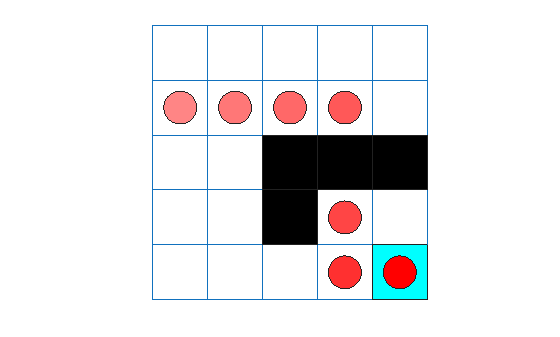The agent trace shows that the agent successfully found the jump from state [2,4] to cell [4,4].

### Create and Train SARSA Agent

To create the SARSA agent using the same Q table representation and epsilon-greedy configuration as the Q-learning agent. For more information on creating SARSA agents, see `rlSARSAAgent` and `rlSARSAAgentOptions`.

```agentOpts = rlSARSAAgentOptions; agentOpts.EpsilonGreedyExploration.Epsilon = 0.04; sarsaAgent = rlSARSAAgent(tableRep,agentOpts);```

Train the SARSA agent using the `train` function. This may take several minutes to complete. To save time while running this example, load a pretrained agent by setting `doTraining` to `false`. To train the agent yourself, set `doTraining` to `true`.

```doTraining = false; if doTraining % Train the agent. trainingStats = train(sarsaAgent,env,trainOpts); else % Load pretrained agent for the example. load('basicGWSARSAAgent.mat','sarsaAgent') end```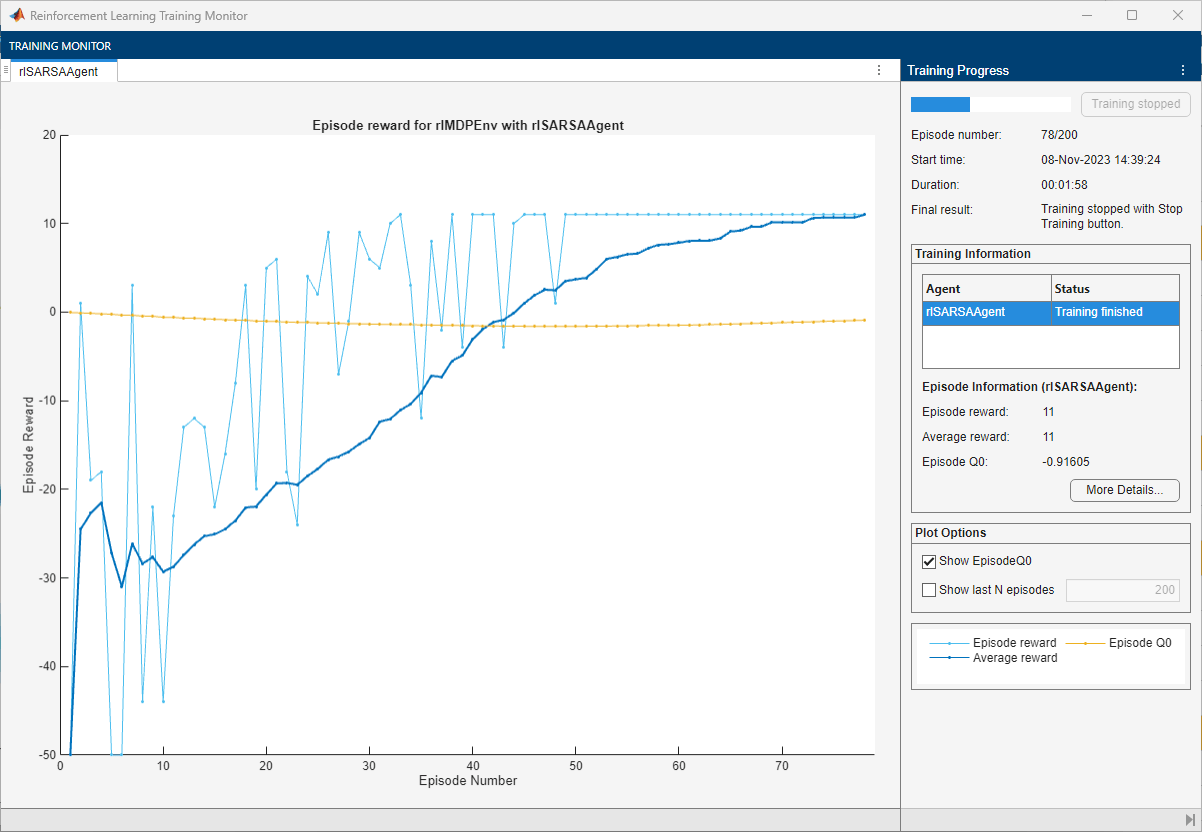### Validate SARSA Training

To validate the training results, simulate the agent in the training environment.

```plot(env) env.Model.Viewer.ShowTrace = true; env.Model.Viewer.clearTrace;```

Simulate the agent in the environment.

`sim(sarsaAgent,env)`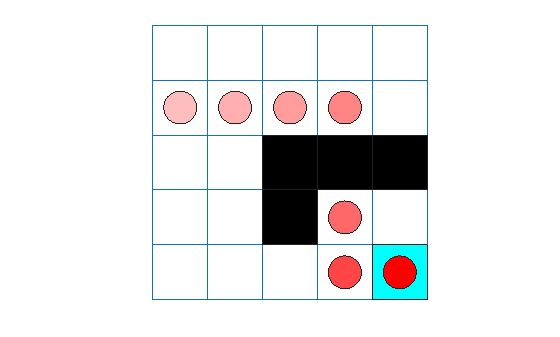The SARSA agent finds the same grid world solution as the Q-learning agent.